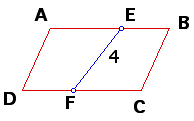# Slicing a Parallelogram

Suppose AB =10 in the parallelogram ABCD below. If a point E is on AB and a point F is on CD such that EF divides that area of ABCD in half, what is FD if EF = 4?If EF = 4, what is the area of the parallelogram? (Remember to take into account all the possible cases.)